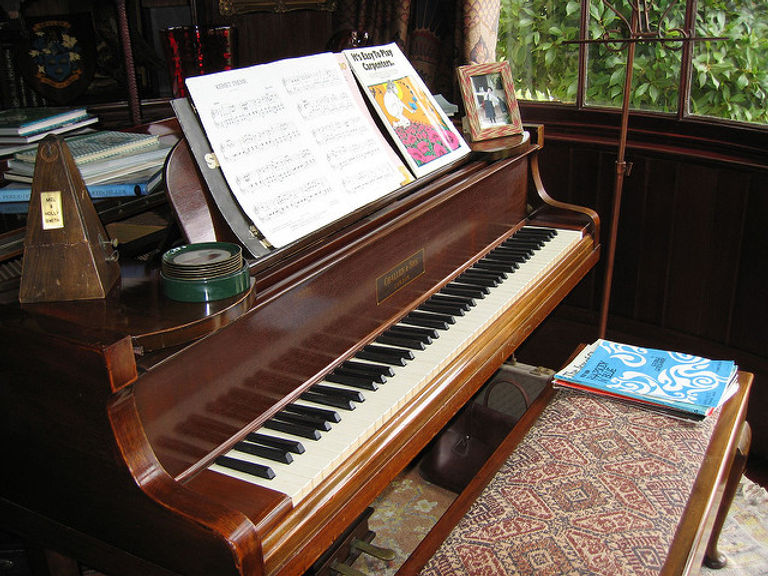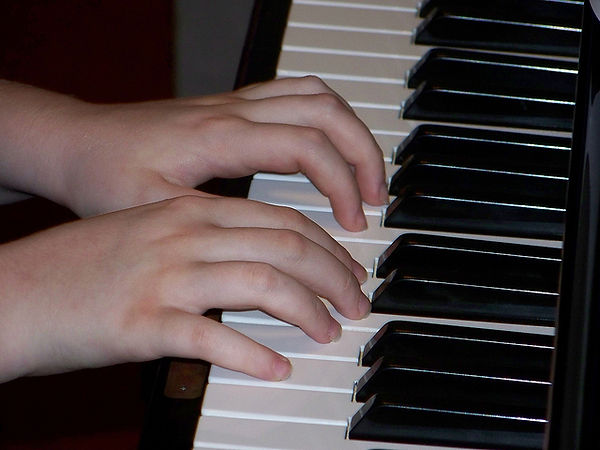top of page# Learn Keyboard Bernardsville

## Major Scales

Here is how you can learn keyboard major scales. On the piano keyboard:

• A half-step is the distance from one key to the next. It may be a white key, or it may be a black key.

• A whole-step is made up of two half-steps.

A Major Scale is built on a pattern of

• whole-step, whole-step, half-step

• whole-step, whole-step, whole-step, half-step## Learn Keyboard Intervals

SECOND

From C to D is the interval of a 2nd.

THIRD

From C to E is the interval of a 3rd. E is the middle tone of a major chord.

FOURTH

From C to F is the interval of a 4th.

FIFTH

From C to G is the interval of a 5th.

SIXTH

From C to A is the interval of a 6th. This is one tone above the 5th.

SEVENTH

From C to B is a 7th.

EIGHTH (OCTAVE)

From C to C is the interval of an octave. This is the same tone eight notes higher.## Build a Major Chord

An example of a simple chord is the triad. A triad is made of three tones which are sounded at the same time. In the key of C Major, the below example is a first triad built (chord) on C.

Put your left finger on C, and with your right hand count up 4 half-steps. ( A half-step is the distance from one key to the next. It doesn't matter whether that key is black or white.) So we start going up by half-steps; C#, D, D#, E, (1,2,3,4). We have gone up 4 half-steps and we are now on E. E is the next note of our triad.

Now hold down the E with your left finger and count up 3 half-steps with your right hand; F, F#, G, (1,2,3). You are now on G and G is the top note of the triad.

Play the three notes together; C,E,G. This is the C Major chord or triad in root position. C is the low (bottom) note of the chord.

All major chords in root position will have this pattern of 4 half-steps and 3 half-steps. If the bottom note is on a line, the chord will be line-line-line. If the bottom note is on a space, the chord will be space-space-space.## Chord Numbers and Inversions

As you learn piano, you can build a major chord on each note of the scale. We use Roman numerals to mark them.

• If the chord is built on the first note of the scale, it is a I (One) chord.

• If the chord is built on the 4th note of the scale, we call it a IV (Four) chord.

• If it is built on the 5th note of the scale, it is a V (Five) chord.

In C Major, C is the first note of the scale, so we build the I (One) chord on C.

• F is the 4th note of the scale, so we build the IV (Four) chord on F.

• G is the 5th note of the scale, so let's build the V (Five) chord on G.

After we build the chords in root position , we can change the notes around.

Instead of a C,E,G chord, we can put the E as the bottom note and we now have E,G,C as the tones of the chord. We call this the first inversion of the chord.

Put the G on the bottom to make a G,C,E chord and this is the second inversion of the chord.## The V7 Chord

When you build a V (Five) chord in C Major, the bottom note is G because G is the 5th note of the C Major scale. We know that the G chord is made up of the notes G,B, and D. Now if you add the 7th tone of the scale, F, you have a nice sound for harmony. We can invert the chord and sometimes we leave out one of the tones.

To move from the I (One) chord to the V7 (Five-seven) chord:

• keep the top note the same

• move the middle note up one-half step

• move the bottom note down one-half step## Chord Progressions

In this section of piano lessons, we will learn the chord progression I (One), IV (Four), I, V7 (Five-Seven), I. This series of chords if very useful when harmonizing songs.

In the key of C Major, the I (One) chord is C,E,G. This is the root position. Play this chord.

Keep the bottom note where it is. Move the middle tone up one-half step, and move the top tone up two half-steps (one whole step). Now you are playing C,F,A. This is the IV (Four) chord (second inversion).

Go back to the I chord in root position; C,E,G.

Now move to the V7 (Five-Seven) chord by keeping the top note in place and move the middle tone up one-half step, and the bottom tone down one-half step.

Now go back to the I chord in root position.

First play it with the left hand, then with the right hand, and then with both hands.